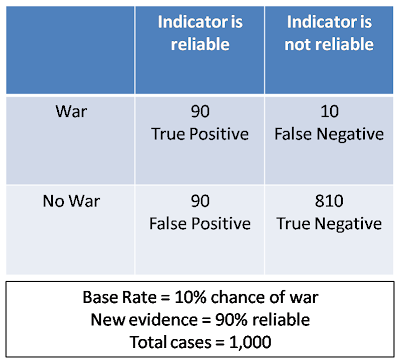## Wednesday, May 6, 2009

### Summary Of Findings: Bayesian Analysis (4 out of 5 Stars)

Note: This post represents the synthesis of the thoughts, procedures and experiences of others as represented in the 12 articles read in advance of (see previous posts) and the discussion among the students and instructor during the Advanced Analytic Techniques class at Mercyhurst College on 6 MAY 2009 regarding Bayesian Analysis specifically. This technique was evaluated based on its overall validity, simplicity, flexibility and its ability to effectively use unstructured data.

Description:
Bayesian analysis is a method that uses Bayesian statistics to assess the likelihood of an event happening in light of new evidence. It generates an estimate and the use of Bayesian statistics in Intelligence analysis allows for the uncertainty of the traditional intelligence data set to be understood in a scientifically valid manner.

Strengths:
*can limit analyst biases by reducing the weight of evidence simply because it is new or vivid
*forces the analyst to resassess evidence and consider alternative possibilites
*provides a numerical likelihood
*provides audit trail and ability to reproduce results

Weaknesses:
*Probabilities are based largely on subjectivity
*Susceptible to biases
*Highly complex problems require heavy computations
*Can be mathematically complex
*Not always useful as a stand alone method (works well in tandem with methods like Delphi); may require SMEs for determining probability distributions
*Some reliance on ambiguous validities
*"Negative evidence"--absence of positive evidence

How-To:

This method loosely follows the guidance suggested by his line of research into the use of natural frequencies in teaching and explaining Bayes to beginners.

1.) Create a 2x2 matrix. Label the quadrants with the respective information that creates true positive, false negative, false positive, and true negative quadrants.
2.) Take the given information, the base line (for example, 100 out of 1,000) with the new information (for example, a new document that is 90% credible saying that war is immiment) which means that your true positive and your false negative must equal 100 and the false positive and true negative must equal 900.
3.) To calculate the true positive quandrant, take 90% of the 100 from the base line (which equals 90).
4.) To calculate the false negative quadrant, take the numerator of the base line (100) and subtract the true positive quadrant (90), creating an answer of 10.
5.) To calculate the true negative quadrant, take 90% of your non-war cases (900), equalling 810.
6.) To calculate the false positives, subtract the sum of the three quadrants known from the total number of cases (1,000), which equals 90.
7.) To calculate the new probablitiy, divide what the numerator of the base line (100) from the new total of positive caes (90+90=180), which equals 55.5%

The 55.5% means that there is a 55% probability that countries X and Y are likely to go to war.Experience:
To understand the basic mathematical principles behind Bayes, the class worked through some sample problems. One of the problems was based on a medical test with an 80% accuracy rate for a cancer with 2% affliction rate in the general population. The class applied this to a sample population of 1000 cases. We established a matrix and assessed the true positive, false positive, false negative, and true negative quantities (16, 116, 4, and 784 respectively). We plugged these numbers into the appropriate matrix fields. We then divided the number of actual cases of cancer (20 or 2% of 1000) into the number of positive tests (132--the 16 true positives and 116 false postives). The result was 15% rate of those who have the cancer from the positive tests, a rather stark difference from the 2% base rate! This problem actually reflects the number of breast cancer rates from a medical treatment from around a two decades ago!
Note: see the matrix for a synopsis of another of the problems we worked through (a peice of evidence emerging suggesting a cause for war).

The class also used a Bayesian application to assess the likelihood we would contract swine flu. We started with the initial hypothesis that we would contract swine flu or we would not contract swine flu, and assigned an initial probability to each hypothesis (the latter >5%) We then added weighted evidence which influenced the base rate of the hypothesis. After all the evidence was entered, the class assessed the likelihood of contracting swine flu.

1.2.